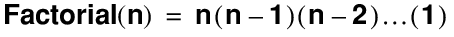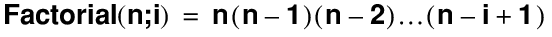Reference > Functions reference > Number functions > Factorial

# Factorial

Returns the factorial of a number, stopping at 1 or at the optional numberOfFactors.

## Format

`Factorial ( number {; numberOfFactors } )`

## Parameters

`number` - numeric expression or field containing a positive integer.

`numberOfFactors` - any numeric expression or field containing a number that represents how many factors to include in the multiplication.

Parameters in braces { } are optional.

number

## Originated in

FileMaker Pro 7.0

## Description

This function is useful in statistics and combinatorics.

Where `n = number` and `i = numberOfFactors`:## Example 1

`Factorial(3)` returns 6, which = 3 * 2 * 1.

`Factorial(10;3)` returns 720, which = 10 * 9 * 8.

## Related topics

Functions reference (category list)

Functions reference (alphabetical list)